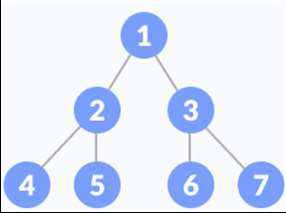# Golang Program to traverse a given tree in Postorder Traversal (Recursive).

## Example

Suppose we have the following binary tree.Postorder Tree Traversal Output − 2 4 5 3 6 7 1.

Approach to solve this problem

Step 1 − If the root node of the given tree is nil, then return; else, follow the steps given below.

Step 2 − Traverse the Left sub-tree.

Step 3 − Traverse the Right sub-tree.

Step 4 − Print the root node data.

## Example

Live Demo

package main
import "fmt"
type Node struct {
data int
left *Node
right *Node
}
func (root *Node)PostOrderTraversal(){
if root !=nil{
root.left.PostOrderTraversal()
root.right.PostOrderTraversal()
fmt.Printf("%d ", root.data)
}
return
}
func main(){
tree := Node{1, &Node{2, &Node{4, nil, nil}, &Node{5, nil, nil}}, &Node{3, &Node{6, nil, nil}, &Node{7, nil, nil}}}
fmt.Printf("Post Order Traversal of the given tree is: ")
tree.PostOrderTraversal()
}

## Output

Post Order Traversal of the given tree is: 4 5 2 6 7 3 1